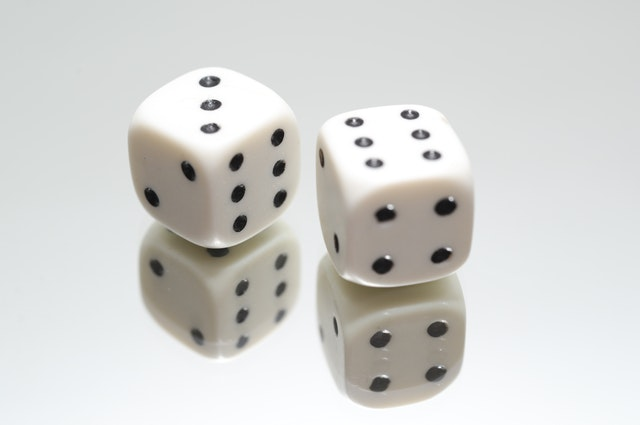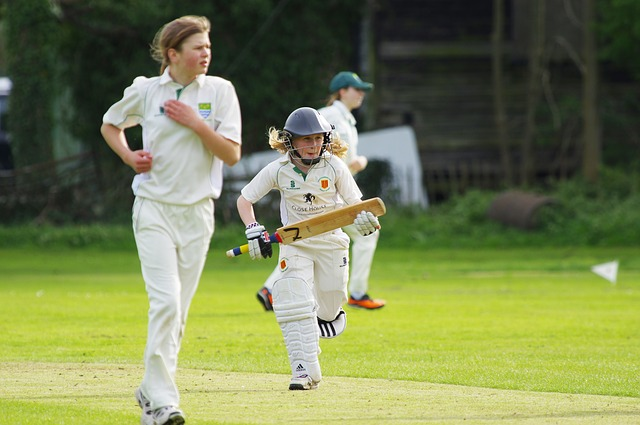## 16.1 Introduction

In this chapter, probability is discussed. In short, probability quantifies the chance that something (an ‘event’) will happen in the future.

More formally, probability is discussed in the context of a procedure whose result is unknown beforehand. A list of all possible results from this procedure is called the sample space. An event is then defined as any combination of these elements of the sample space.

Definition 16.1 (Sample space) The sample space is a list of all possible and distinct results after administering a procedure, whose result is unknown beforehand.
Definition 16.2 (Event) An event is any combination of the elements in the sample space.Example 16.1 (Sample spaces and events) Consider rolling a fair, six-sided die. We do not know what face will be uppermost until we roll the die.

However, the sample space for this procedure can be listed: 1, 2, 3, 4, 5, 6. These are all distinct results (no overlap), and the sample space is discrete.

Many events could be defined using this sample space; for example:

• Rolling a 4: This event includes one element of the sample space: 4.
• Rolling a even number: This event includes three elements of the sample space: 2, 4 and 6.
• Rolling a number larger than 2: This event includes four elements of the sample space: 3, 4, 5 and 6.Example 16.2 (Sample spaces and events) Consider the distance which you can throw a cricket ball. We do not know what distance your throw will be until you throw, but we can describe the sample space for this procedure: the distance could be anywhere between (say) 0 and 200 metres (and of course, some of those distances are fairly unlikely to occur…).

This sample space is continuous.

Many events could be defined using this sample space; for example:

• Throwing more than 50 metres: This event includes elements of the sample space greater than 50m.
• Throwing between 10 and 40 metres: This event includes elements of the sample space between 10m and 40m.
• Throwing less than 20 metres: This event includes elements of the sample space less than 20m.
Because the sample space is continuous, throwing an exact distance (such as exactly 10 metres) is technically not possible.

Once a sample space is defined, a probability can be defined.Definition 16.3 (Probability) A probability is a number between 0 and 1 inclusive (or between 0% and 100% inclusive) that quantifies the likelihood that a certain event will occur.

A probability of zero (or 0%) means the event is ‘impossible’ (will never occur). At the other extreme, a probability of one (or 100%) means that the event is ‘certain’ to happen (will always occur). Most events have a probability between the extremes of 0% and 100%.

Example 16.3 (Probabilities) Consider these cases:

• The probability of receiving negative rainfall is zero: It is impossible.
• The probability of receiving some rain in Buderim in the next decade is one. It is certain.
• The probability of receiving rain on any given day… is somewhere between 0 and 1.

Different ways exist to calculate probabilities of events, including:

• the classical approach (Sect. 16.2);
• the relative frequency approach (Sect. 16.3); and
• the subjective approach (Sect. 16.4).